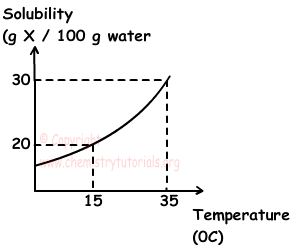## Solutions Exam2 and Problem Solutions

Solutions Exam2 and  Problem Solutions

1. Solubility vs. temperature graph of X solid is given below. Using this graph decide, which ones of the following statements are true;I. When X is dissolved in water, temperature of water decreases.

II. 200 g solution under 35 0C, using 60 g X is saturated solution.

III. When 50 g saturated solution at 35 0C is cooled to 15 0C, 5 g X crystallizes.

Solution:

I. As you can see from the graph, solubility of X in water increases with increasing temperature. Thus, solubility of X in water in endothermic. When X dissolves in closed container, it absorbs heat from water and as a result temperature of water decreases. I is true.

II. At 35 0C;

100 g water dissolves 30 g X

200 g water dissolves ? g X

¯¯¯¯¯¯¯¯¯¯¯¯¯¯¯¯¯¯¯¯¯¯¯¯¯¯

?=60 g X can be dissolved

Since amount of X is 60 g in 200 g solution, it is saturated solution. II is true.

III. 100 water can dissolve 30 g X at 35 0C and 20 g X at 15 0C. When solution prepared under 35 0C is cooled to 15 0C;

30-20=10 X is crystallized.

In 100 g water 10 g X is crystallized

In 50 g water ? g  X is crystallized

¯¯¯¯¯¯¯¯¯¯¯¯¯¯¯¯¯¯¯¯¯¯¯¯¯¯

?=5 g X is crystallized in 50 g water

III is also true.

2. If solubility of sugar in water is endothermic, which ones of the following statements increase both solubility of sugar and solubility rate?

I. Cooling solution

II. Using granulated sugar instead cube sugar

III. Mixing the solution

IV. Increasing amount of sugar

V. Increasing temperature of solution

Solution:

II, III and IV do not affect solubility. In endothermic solutions increasing temperature increases solubility of that matter. Moreover, increasing temperature also increases solubility rate. Thus, V increase both solubility and solubility rate of sugar in water.

3. We add 700 mL water at same temperature to 0,2 molar 300 mL NaCl solution. Find final molarity of this solution.

Solution:

M1=0,2 molar

V1=300 mL

V2=700+300=1000 mL

We use dilution formula;

M1.V1=M2.V2

0,2.300=M2.1000

M2=0,06 molar

4. 9,8 g H2SO4 is dissolved in water and 200 mL solution is prepared. Find normality of solution.(H2SO4=98)

Solution:

There is a relation between normality and molarity;

N=M.Equivalent

nH2SO4=9,8/98=0,1mol H2SO4

M=n/V=0,1/0,2=0,5 molar

V=200 mL=0,2 L

N=M.Equivalent (Where equivalent is 2 since H2SO4 gives 2 H+ ion to solution)

N=0,5.2=1N

5. 0,4 mol MgCl2 and 0,6 mol AlCl3 are dissolved in water and 250 mL solution is prepared. Find molar concentration of [Cl-] in this solution.

Solution:

We write ionization reactions of both salts and find number of moles of ions;

MgCl2(s) → Mg+2(aq) + 2Cl-(aq)

0,4mol        0,4mol        0,8mol

AlCl3(s) → Al+3(aq) + 3Cl-(aq)

0,6mol     0,6mol        1,8mol

Mole of Cl- ion = 0,8 + 1,8 =2,6mol

Volume of Solution=250mL=0,25L

[Cl-]=nCl-/Vsol.=2,6/0,25=10,4molar

Related